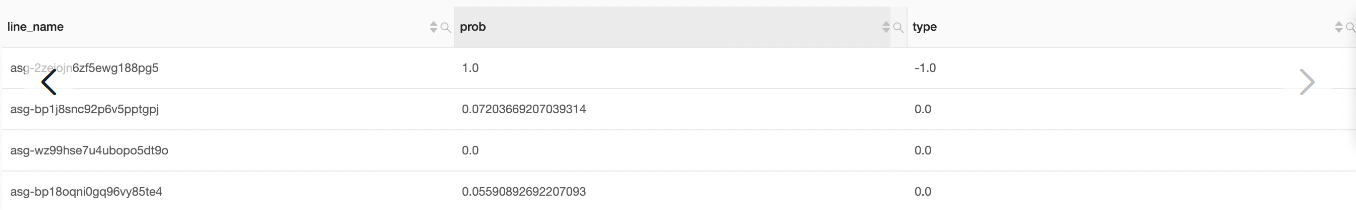This topic describes multi-period estimation functions that you can use to estimate the periodicity of time series data distributed in different time intervals. This topic also describes how to extract the periodicity by using a series of operations such as Fourier transform (FT).

## Function list

Function Description
ts_period_detect Estimates the periodicity of time series data distributed in different time intervals.
ts_period_classify Uses FT to calculate the periodicity of specified time series curves. This function can be used to identify periodic curves.

## ts_period_detect

Function format:
``select ts_period_detect(x,y,minPeriod,maxPeriod)``
The following table lists the parameters in the function.
Parameter Description Value
x The time sequence. Points in time are sorted in ascending order along the horizontal axis. Each point in time is a UNIX timestamp. Unit: seconds.
y The sequence of numeric data at a specific point in time. None.
minPeriod The ratio of the minimum length of a time series data within a period to the total length of the time series data. The ratio is estimated based on your time series curve. The parameter value must be a decimal number. Valid values: (0.0, 1.0].
maxPeriod The ratio of the maximum length of a time series within a period to the total length of the time series data. The ratio is estimated based on your time series curve.
Notice The value of the maxPeriod parameter must be greater than that of the minPeriod parameter. The value must be less than 0.5. If you set the maxPeriod parameter to a value greater than 0.5, the system automatically changes the value to 0.5.
The parameter value must be a decimal number. Valid values: (0.0, 1.0].
Example
• The following query statement is executed:
``* | select ts_period_detect(stamp, value, 0.2, 0.5) from ( select __time__ - __time__ % 120 as stamp, avg(v) as value from log GROUP BY stamp order by stamp )``
• Output result

The output result is of the array type. The result contains UNIX timestamps, statistical values (such as average traffic), and status codes. Each red circle in the following figure represents a status code whose value is 1.0. The following figure shows the output result.

Each shaded part between two consecutive red circles in the following figure represents a period. The curve of each period tends to be the same.## ts_period_classify

Function format:
``select ts_period_classify(stamp,value,instanceName)``
The following table lists the parameters in the function.
Parameter Description Value
stamp The time sequence. Points in time are sorted in ascending order along the horizontal axis. Each point in time is a UINX timestamp. Unit: seconds.
value The sequence of numeric data at a specific point in time. None.
instanceName The name of the time series curve. None.
Example:
• The following query statement is executed:
``* and h : nu2h05202.nu8 | select ts_period_classify(stamp, value, name) from log``
• ResponseThe following table lists the display items.
Display item Description
line_name The name of the time series curve.
prob The ratio of the number of values within the primary period to the total number of values on the time series curve. Valid values: [0, 1]. You can set the value to 0.15 for testing.
type The type of the time series curve. Valid values: -1, -2, and 0.
• The value -1 indicates that the length of the time series curve is too short (less than 64 points).
• The value -2 indicates that the time series curve has a high failure rate (higher than 20%).
• The value 0 indicates that the time series curve is periodic.